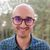# QlikView App Dev

Discussion Board for collaboration related to QlikView App Development.

Announcements
Become an analytics expert with Qlik's new 15 week course: Applied Data Analytics using Qlik Sense. READ MORE
cancel
Showing results for
Did you mean:Contributor III

## qlikview

Hi, I need to get one extra column as GetPercentage. This one I need to get by dividing row4/row7 etc. As there are other rows which also I need, this is an example. How to achieve this in Qlikview load script or expression. Thanks in Advance.

Attached is the pic image

Labels (3)

• ### QlikView

1 Solution

Accepted SolutionsContributor III
Author
There are few columns, what I need eg: RowNo Column : row 4(Total4) /ColumnG(Total\$)ie row 7 :

(384144.15/634425.14)*100 I need to get 60.55 next to the row4 Total Column. Pls see the image for reference. Tks! in Advance

5 RepliesMVP

How are the rows selected for the percentage?

Logic will get you from a to b. Imagination will take you everywhere. - A EinsteinPartner
I guess that in in Front end you should you Pivot or Straight table.
In expressions:
1. Label Accommodation, expression = Sum({<Description = {'ACCOMMODATION'}>} Total\$)
2. Label Sales, expression = Sum({<Description = {'SALES-CASH'}>} Total\$)
3. Label Revenue, expression = Sum({<Description = {'TOTAL REVENUE'}>} Total\$)
4. Label Accommodation %, expression = Accommodation/Sales
5. Label Sales %, expression = Sales/Revenue

Is it what you wish to achieve?Contributor III
Author
There are few columns, what I need eg: RowNo Column : row 4(Total4) /ColumnG(Total\$)ie row 7 :

(384144.15/634425.14)*100 I need to get 60.55 next to the row4 Total Column. Pls see the image for reference. Tks! in AdvanceSpecialist II

May be this

Sum(totals)/below(total Sum(totals),3)Contributor III
Author
I was able to resolve using set analysis. Thanks! all for the advise
=100*Sum(Count)/
If(Match(Class, 'SALES-CASH', 'DOCTOR CHARGE'), Sum(TOTAL {<Class = {'TOTAL REVENUE'}>} Count),
If(Class = 'SURGICALS & CONSUM', Sum(TOTAL {<Class = {'TOTAL COST OF SALES'}>}Count)
Services'}>}Count),
))